# RD Sharma Solutions Class 7 Chapter 1 Integers

Read RD Sharma Solutions Class 7 Chapter 1 Integers below, students should study RD Sharma class 7 Mathematics available on Studiestoday.com with solved questions and answers. These chapter wise answers for class 7 Mathematics have been prepared by teacher of Grade 7. These RD Sharma class 7 Solutions have been designed as per the latest NCERT syllabus for class 7 and if practiced thoroughly can help you to score good marks in standard 7 Mathematics class tests and examinations

Exercise 1.1

Question 1: Determine each of the following products:

(i) 12 × 7

(ii) (-15) × 8

(iii) (-25) × (-9)

(iv) 125 × (-8)

Solution 1:

(i) 12 × 7

For find the products of following numbers: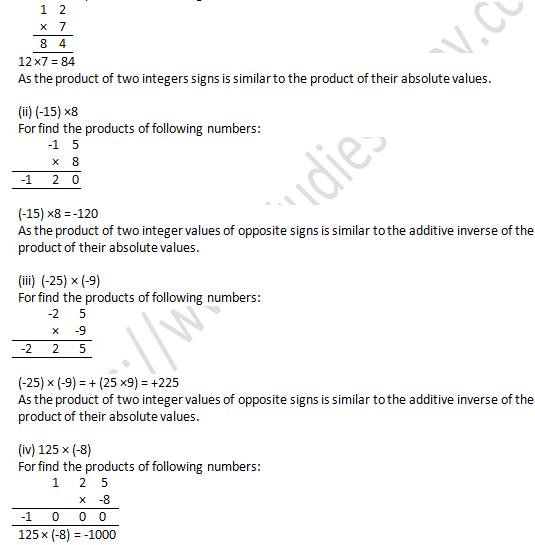As the product of two integer values of opposite signs is similar to the additive inverse of the product of their absolute values.

Question 2.  Find each of the following products:

(i) 3 × (-8) × 5

(ii) 9 × (-3) × (-6)

(iii) (-2) × 36 × (-5)

(iv) (-2) × (-4) × (-6) × (-8)

Solution 2:

(i) 3 × (-8) ×5

For find the products of following numbers:

= 3 × (-8) × 5

= 3 × (-8 × 5)

= 3 × -40

= -120

The product of integers with opposite signs is equivalent to the additive inverse of the product of their absolute values.

(ii) 9 × (-3) × (-6)

For find the products of following numbers:

= 9 × (-3) × (-6)

= 9 × (-3 × -6)

= 9 × (+18)

= 162

[∵ The product of integers of like signs is equivalent to the product of their absolute values.]

(iii) (-2) × 36 × (-5)

For find the products of following numbers:

= (-2) × 36 × (-5)

= (-2 × 36) × (-5)

= -72 × -5 = +360

[∵ The product of integers of like signs is equivalent to the product of their absolute values.]

(iv) (-2) × (-4) × (-6) × (-8)

For find the products of following numbers:

= (-2) × (-4) × (-6) × (-8)

= (-2 × -4) × (-6 × -8)

= -8 × (-48) = +384

[∵ The product of integers of like signs is equivalent to the product of their absolute values.]

Question 3. Find the value of:

(i) 1487 × 327 + (-487) × 327

(ii) 28945 × 99 – (-28945)

Solution 3:

(i) 1487 × 327 + (-487) × 327

According to the multiplication of integers,

1487 × 327 + (-487) × 327

= (1487 × 327) + (-487 × 327)

= 486249 – 159249

=327000

Since the product of integers of opposite signs is equivalent to the additive inverse of the product of their absolute values.

(ii) 28945 × 99 – (-28945)

According to the multiplication of integers,

28945 × 99 – (-28945)

= (28945 × 99) – (-28945)

= 2865555 + 28945

=2894500

Since the product of integers of like signs is equivalent to the product of their absolute values.

Question 4 : Complete the following multiplication table:Is the multiplication table symmetrical about the diagonal joining the upper left corner to the lower right corner?

Solution 4:This is clear from the table that the table is symmetrical also with diagonal that links the upper left corner to the bottom right corner.

Question 5: Determine the integer whose product with ‘-1’ is

(i) 58

(ii) 0

(iii) -225

Solution 5:

(i) 58

For find the integer of which is multiplied by -1

= 58 × (-1) = -58

Since the product of integers of opposite signs is equivalent to the additive inverse of the product of their absolute values.

(ii) 0

For find the integer of which is multiplied by -1

= 0 × -1 = 0

[because when any digit  multiplied with 0 we get 0 as their result]

(iii) -225

For find the integer of which is multiplied by -1

= -225 × -1 = 225

Since the product of integers of like signs is equivalent to the product of their absolute values.

Exercise 1.2

Question 1. Divide:

(i) 102 by 17

(ii) -85 by 5

(iii) -161 by -23

(iv) 76 by -19

(v) 17654 by -17654

(vi) (-729) by (-27)

(vii) 21590 by -10

(viii) 0 by -135

Solution 1 :Exercise 1.3

Question 1: Find the value of

1. 36 ÷ 6 + 3

Solution 1:

According to BODMAS rule

we have to division first: 36 ÷ 6 = 6

then we have to do addition: 6 + 3 = 9

Question 2: 24 + 15 ÷ 3

Solution 2:

According to BODMAS rule

we have to division first: 15 ÷ 3 = 5

then we have to do addition: 5 + 24 = 29

Question: 3. 120 – 20 ÷ 4

Solution 3:

According to BODMAS rule

we have to division first: 20 ÷ 4 = 5

then we have to do subtraction: 120 – 5 = 115

Question: 4. 32 – (3 × 5) + 4

Solution 4:

According to BODMAS rule

we have to multiplication first: 3 × 5 =15

then addition: 15 ­+ 4 = 19

then subtraction: 32 – 19 = 21

Question: 5. 3 – (5 – 6 ÷ 3)

Solution 5:

According to BODMAS rule

we have to division first: 6 ÷ 3 = 2

then subtraction: (5 – 2) = 3

then subtraction:3 – 3 = 0

Question: 6. 21 – 12 ÷ 3 × 2

Solution 6:

According to BODMAS rule

we have to division first: 12 ÷ 3= 4

then multiplication: 4 × 2 =

then subtraction: 21 – 8 = 13

Question: 7. 16 + 8 ÷ 4 – 2 × 3

Solution 7:

According to BODMAS rule

we have to division first: 8 ÷ 4 = 2

then multiplication: 2 × 3 = 6

then addition: 16 + 2= 18

then subtraction: 18 – 6 = 12

Question: 8. 28 – 5 × 6 + 2

Solution 8:

According to BODMAS rule

we have to multiplication first: 5 × 6= 30

then addition: -30 + 2 = -28

then subtraction: 28 – 28 = 0

Question: 9. (-20) × (-1) + (-28) ÷ 7

Solution 9:

According to BODMAS rule

we have to division first:

then multiplication:

then subtraction:

Therefore, (-20) × (-1) + (-28) ÷ 7 = (-20) × (-1) – 4

= 20 – 4 = 16

Question: 10. (-2) + (-8) ÷ (-4)

Solution 10:

According to BODMAS rule

we have to division first: (-8) ÷ (-4) = 2

then addition: (-2) + 2 = 0

Question: 11. (-15) + 4 ÷ (5 – 3)

Solution 11:

we have to solve the bracket first: (5 – 3) = 2

then division: 4 ÷ 2= 2

then addition: ( -15) + 2 = -13

Question: 12. (-40) × (-1) + (-28) ÷ 7

Solution 12:

According to BODMAS rule

we have to division first: (-28) ÷ 7= -4

then multiplication: (-40) × (-1)= 40

then addition: 40 + (-4) = 36

Question: 13. (-3) + (-8) ÷ (-4) -2 × (-2)

Solution 13:

According to BODMAS rule

we have to division first: (-8) ÷ (-4) = 2

then multiplication: -2 × (-2) = 4

then addition: 2 + 4 = 6

then subtraction: 6 -3 = 3

Question: 14. (-3) × (-4) ÷ (-2) + (-1)

Solution 14:

(-3) × (-4) ÷ (-2) + (-1)

According to BODMAS rule

We have to division first: (-4) ÷ (-2) = 2

Then multiplication: (-3) × 2 = -6

Then addition: (-6) + (-1) = -6 – 1 = -7

Exercise 1.4

Question 1: Simplify each of the following:

1. 3 – (5 – 6 ÷ 3)

Solution 1:

According to BODMAS rule firstly, solve the bracket:

3 – (5 – 6 ÷ 3)

= 3 – (5 – 2)

= 3 – 3

= 0

Question 2: -25 + 14 ÷ (5 – 3)

Solution 2:

According to BODMAS rule firstly, solve the bracket:

-25 + 14 ÷ (5 – 3)

= -25 + 14 ÷ 2

= -25 + 7

= -18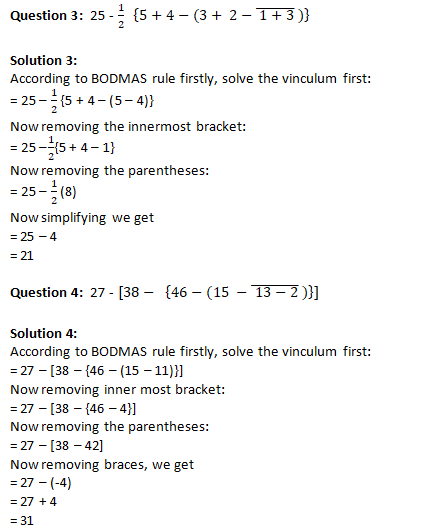Question 5:  36 - [18 - {14- (15 - 4 ÷ 2 x 2)}]

Solution 5:

According to BODMAS rule firstly, solve the inner most bracket first:

= 36 – [18 – {14 – (11 ÷ 2 × 2)}]

= 36 – [18 – {14 – 11}]

Now removing the parentheses we get

= 36 – [18 – 3]

Now remove the braces we get

= 36 – 15

= 21

Question: 6. 45 – [38 – {60 ÷ 3 – (6 – 9 ÷ 3) ÷ 3}]

Solution 6:

According to BODMAS rule firstly, solve the inner most bracket first:

= 45 – [38 – {20 – (6 – 3) ÷ 3}]

= 45 – [38 – {20 – 3 ÷ 3}]

Now remove the parentheses:

= 45 – [38 – 19]

Now remove the braces:

= 45 – 19

= 26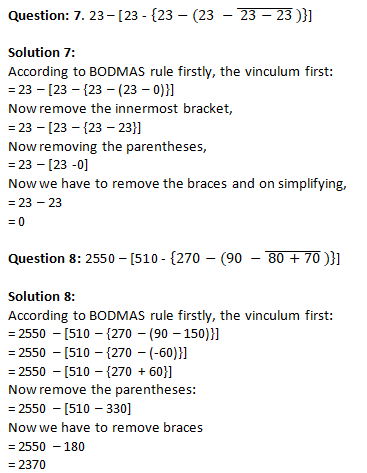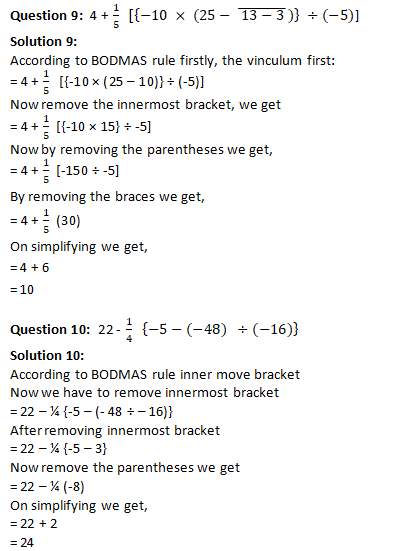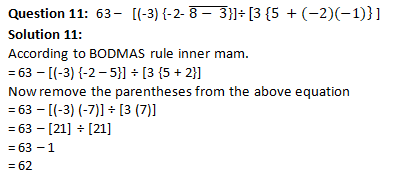Question 12:  [29 – (-2) { 6 – (7 – 3)}] ÷ [ 3 × {5 + (-3) × (-2)]}

Solution 12:

According to BODMAS rule inner move bracket

First we have to remove the innermost brackets,

= [29 – (-2) {6 – 4}] ÷ [3 × {5 + 6}]

Now remove the parentheses ,

= [29 + 2 (2)] ÷ [3 × 11]

Now remove all braces present,

= 33 ÷ 33

= 1

Question: 13. Using brackets, write a mathematical expression for each of the following:

(i) Nine multiplied by the sum of two and five.

(ii) Twelve divided by the sum of one and three.

(iii) Twenty divided by the difference of seven and two.

(iv) Eight subtracted from the product of two and three.

(v) Forty divided by one more than the sum of nine and ten.

(vi) Two multiplied by one less than the difference of nineteen and six.

Solution 13:

(i) 9 × (2 + 5)

(ii) 12 ÷ (1 + 3)

(iii) 20 ÷ (7 – 2)

(iv) 2 × 3 -8

(v) 40 ÷ [1 + (9 + 10)]

(vi) 2 × [(19 -6) -1]